# 十一、通过人工神经网络的机器学习

• 机器学习ML）和人工神经网络ANN）之间的区别
• 多层感知器MLP）网络
• 如何定义和实现 MLP 网络
• 评估和改善我们的人工神经网络
• 如何使用经过训练的 ANN 识别图像中的对象

# 机器学习（ML）与人工神经网络（ANN）

ANN 什么时候有用？ 想象一下，我们已经实现了一个对象识别器，该对象识别器经过训练可以识别背包和鞋子，然后得到以下图像：SVM 还可以使用称为核技巧的东西执行非线性分类，并将输入隐式映射到高维特征空间。

# ANN 如何工作？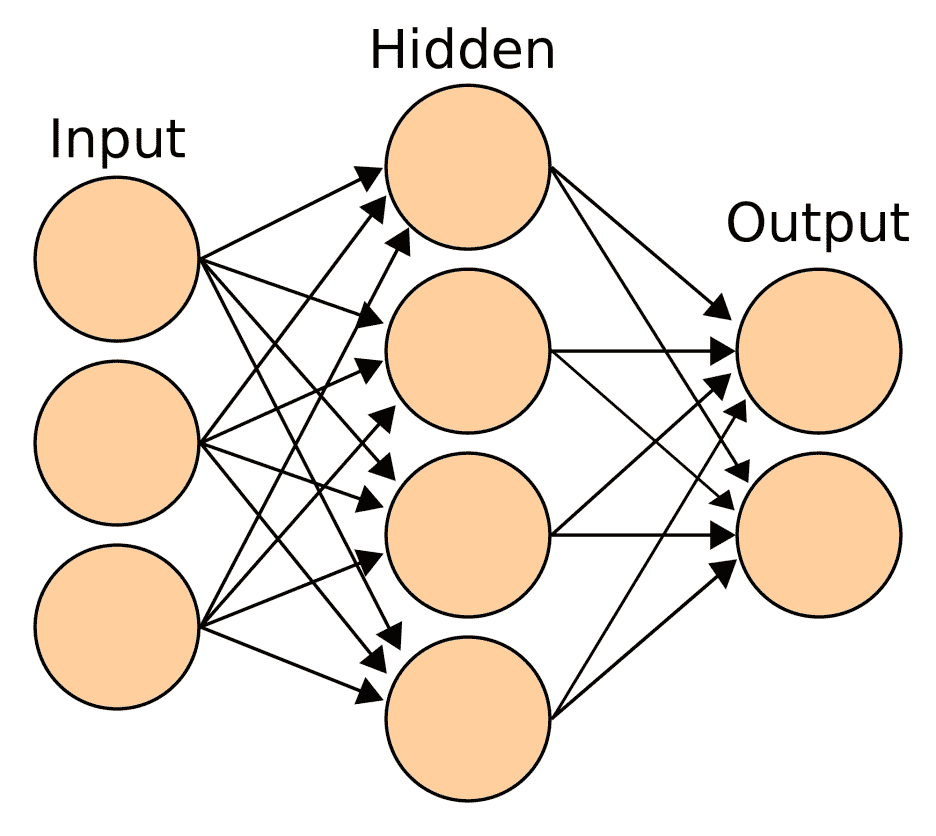MLP 网络至少由三层组成：

• 输入层：每个 MLP 始终具有这些层之一。 它是一个被动层，这意味着它不会修改数据。 它从外界接收信息，并将其发送到网络。 该层中节点（神经元）的数量将取决于我们要从图像中提取的特征或描述性信息的数量。 例如，在使用特征向量的情况下，向量中的每一列将有一个节点。
• 隐藏层：所有基础工作都在此层进行。 它将输入转换为输出层或另一个隐藏层可以使用的东西（可以有多个）。 该层充当黑匣子，在接收到的输入中检测模式并评估每个输入的权重。 其行为将由其激活函数提供的方程式定义。
• 输出层：此层也将始终存在，但是在这种情况下，节点数将由所选的神经网络定义。 该层可能具有三个神经元。 输出层可以由单个节点构建（线性回归），也就是说，我们想知道图像是否带有背包。 但是在进行多类分类的情况下，该层将包含几个可以识别的节点，每个对象一个。 默认情况下，每个节点都会产生一个值，该值的范围为[-1,1]，该值定义对象是否存在的可能性，并允许在单个输入图像上进行多类检测。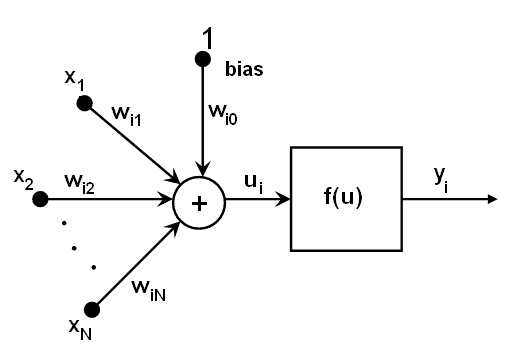# 如何定义多层感知器（MLP）

MLP 是 ANN 的一个分支，由于其能够在嘈杂或意外环境中识别模式，因此广泛用于模式识别。 MLP 可用于实现有监督和无监督的学习（在第 9 章，“对象识别”中都对它们进行了讨论）。 除此之外，MLP 还可以用于实现另一种学习，例如受行为心理学启发的强化学习，其中使用奖励/惩罚行为来调整网络学习。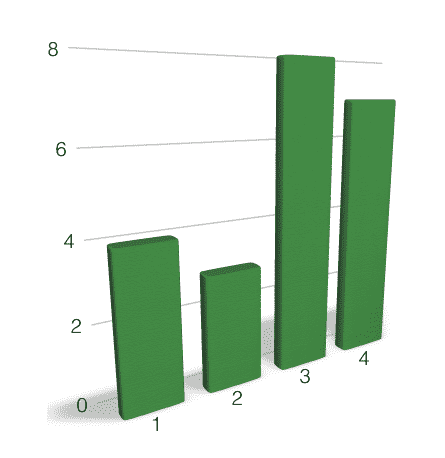• 隐藏神经元的数量应在输入层的大小和输出层的大小之间
• 隐藏神经元的数量应为输入层大小的三分之二加上输出层大小的三分之二
• 隐藏神经元的数量应小于输入层大小的两倍

# 如何实现 ANN-MLP 分类器？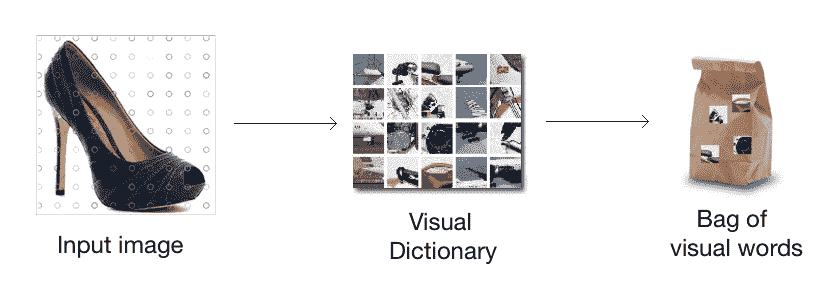$python create_features.py --samples bag images/bagpack/ --samples dress images/dress/ --samples footwear images/footwear/ --codebook-file models/codebook.pkl --feature-map-file models/feature_map.pkl  feature_map.pkl文件中，我们拥有训练期间将参与的每个图像的特征向量。 首先，我们将为 ANN 分类器创建一个类，在其中设置网络层的大小： from sklearn import preprocessing import numpy as np import cv2 import random class ClassifierANN(object): def __init__(self, feature_vector_size, label_words): self.ann = cv2.ml.ANN_MLP_create() self.label_words = label_words # Number of centroids used to build the feature vectors input_size = feature_vector_size # Number of models to recongnize output_size = len(label_words) # Applying Heaton rules hidden_size = (input_size * (2/3)) + output_size nn_config = np.array([input_size, hidden_size, output_size], dtype=np.uint8) self.ann.setLayerSizes(np.array(nn_config)) # Symmetrical Sigmoid as activation function self.ann.setActivationFunction(cv2.ml.ANN_MLP_SIGMOID_SYM, 2, 1) # Map models as tuples of probabilities self.le = preprocessing.LabelBinarizer() self.le.fit(label_words) # Label words are ['dress', 'footwear', 'backpack']  作为输出，我们决定用二进制数[0,0,1][0,1,0][1,0,0]实现一个概率元组，目的是通过这种方式获得多类检测。 作为激活函数对称 Sigmoid（NN_MLP_SIGMOID_SYM），这是 MLP 的默认选择，其中输出将在[-1,1]范围内。 这样，我们的网络生成的输出将定义概率而不是分类结果，从而能够识别同一样本图像中的两个或三个对象。 在训练过程中，我们将数据集分为两个不同的集：训练和测试。 我们将为其定义一个比率（通常，大多数示例建议使用 75% 作为训练集，但可以对其进行调整，直到获得最佳准确率为止），并随机选择项目以防止偏差。 这是如何运作的？ class ClassifierANN(object): ... def train(self, training_set): label_words = [ item['label'] for item in training_set] dim_size = training_set['feature_vector'].shape train_samples = np.asarray( [np.reshape(x['feature_vector'], (dim_size,)) for x in training_set] ) # Convert item labels into encoded binary tuples train_response = np.array(self.le.transform(label_words), dtype=np.float32) self.ann.train(np.array(train_samples, dtype=np.float32),cv2.ml.ROW_SAMPLE, np.array(train_response, dtype=np.float32) )  在这种情况下，我们对输入层的每个节点使用了相同的权重（默认行为），但是我们可以指定它们为特征向量中的列提供更多权重，并提供更多重要信息。 # 评估训练后的网络 为了评估我们训练有素的 MLP 网络的鲁棒性和准确率，我们将计算混淆矩阵（也称为误差矩阵）。 该矩阵将描述我们分类模型的表现。 混淆矩阵的每一行代表预测类中的实例，而每一列代表实际类中的实例（反之亦然）。 为了填充矩阵，我们将使用测试集对其进行评估： from collections import OrderedDict def init_confusion_matrix(self, label_words): confusion_matrix = OrderedDict() for label in label_words: confusion_matrix[label] = OrderedDict() for label2 in label_words: confusion_matrix[label][label2] = 0 return confusion_matrix # Chooses the class with the greatest value, only one, in the tuple(encoded_word) def classify(self, encoded_word, threshold=0.5): models = self.le.inverse_transform(np.asarray([encoded_word]), threshold) return models # Calculate the confusion matrix from given testing data set def get_confusion_matrix(self, testing_set): label_words = [item['label'] for item in testing_set] dim_size = testing_set['feature_vector'].shape test_samples = np.asarray( [np.reshape(x['feature_vector'], (dim_size,)) for x in testing_set] ) expected_outputs = np.array(self.le.transform(label_words), dtype=np.float32) confusion_matrix = self._init_confusion_matrix(label_words) retval, test_outputs = self.ann.predict(test_samples) for expected_output, test_output in zip(expected_outputs, test_outputs): expected_model = self.classify(expected_output) predicted_model = self.classify(test_output) confusion_matrix[expected_model][predicted_model] += 1 return confusion_matrix  作为样本混淆矩阵，并考虑一个包含 30 个元素的测试集，我们可能获得以下结果： 鞋子 背包 连衣裙 鞋子 8 2 0 背包 2 7 1 连衣裙 2 2 6 考虑到先前的矩阵，我们可以通过以下公式计算训练网络的准确率：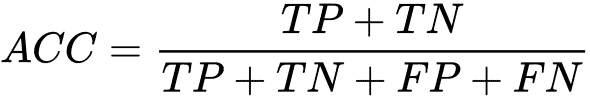在此公式中，我们表示真正例TP），真负例（TN），假正例FP）和假负例FN）。 就鞋类而言，我们可以说其准确率为 80%。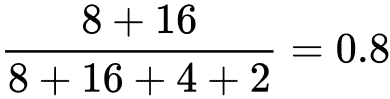上式的实现代码如下： def calculate_accuracy(confusion_matrix): acc_models = OrderedDict() for model in confusion_matrix.keys(): acc_models[model] = {'TP':0, 'TN':0, 'FP':0, 'FN': 0} for expected_model, predicted_models in confusion_matrix.items(): for predicted_model, value in predicted_models.items(): if predicted_model == expected_model: acc_models[expected_model]['TP'] += value acc_models[predicted_model]['TN'] += value else: acc_models[expected_model]['FN'] += value acc_models[predicted_model]['FP'] += value for model, rep in acc_models.items(): acc = (rep['TP']+rep['TN'])/(rep['TP']+rep['TN']+rep['FN']+rep['FP']) print('%s \t %f' % (model,acc))  收集本节中的每个代码块，我们已经实现了ClassifierANN类以供使用： ############### # training.py ############### import pickle def build_arg_parser(): parser = argparse.ArgumentParser(description='Creates features for given images') parser.add_argument("--feature-map-file", dest="feature_map_file", required=True, help="Input pickle file containing the feature map") parser.add_argument("--training-set", dest="training_set", required=True, help="Percentage taken for training. ie 0.75") parser.add_argument("--ann-file", dest="ann_file", required=False, help="Output file where ANN will be stored") parser.add_argument("--le-file", dest="le_file", required=False, help="Output file where LabelEncoder class will be stored") if __name__ == '__main__': args = build_arg_parser().parse_args() # Load the Feature Map with open(args.feature_map_file, 'rb') as f: feature_map = pickle.load(f) training_set, testing_set = split_feature_map(feature_map, float(args.training_set)) label_words = np.unique([item['label'] for item in training_set]) cnn = ClassifierANN(len(feature_map['feature_vector']), label_words) cnn.train(training_set) print("===== Confusion Matrix =====") confusion_matrix = cnn.get_confusion_matrix(testing_set) print(confusion_matrix) print("===== ANN Accuracy =====") print_accuracy(confusion_matrix) if 'ann_file' in args and 'le_file' in args: print("===== Saving ANN =====") with open(args.ann_file, 'wb') as f: cnn.ann.save(args.ann_file) with open(args.le_file, 'wb') as f: pickle.dump(cnn.le, f) print('Saved in: ', args.ann_file)  您可能已经注意到，我们已经将 ANN 保存到两个单独的文件中，因为ANN_MLP类具有自己的保存和加载方法。 我们需要保存用于训练网络的label_words。 Pickle 为我们提供了对对象结构进行序列化和反序列化以及从磁盘保存和加载它们的功能，除了ann这样的结构有自己的实现。 运行以下命令以获取模型文件。 混淆矩阵和准确率概率将与其一起显示： $ python training.py --feature-map-file models/feature_map.pkl --training-set 0.8 --ann-file models/ann.yaml --le-file models/le.pkl


# 图片分类

class FeatureExtractor(object):
def get_feature_vector(self, img, kmeans, centroids):
return Quantizer().get_feature_vector(img, kmeans, centroids)


###############
# classify_data.py
###############

import argparse
import _pickle as pickle

import cv2
import numpy as np

import create_features as cf

# Classifying an image
class ImageClassifier(object):
def __init__(self, ann_file, le_file, codebook_file):
with open(ann_file, 'rb') as f:
with open(le_file, 'rb') as f:

with open(codebook_file, 'rb') as f:

def classify(self, encoded_word, threshold=None):
models = self.le.inverse_transform(np.asarray(encoded_word), threshold)
return models

# Method to get the output image tag
def getImageTag(self, img):
# Resize the input image
img = cf.resize_to_size(img)
# Extract the feature vector
feature_vector = cf.FeatureExtractor().get_feature_vector(img, self.kmeans, self.centroids)
# Classify the feature vector and get the output tag
retval, image_tag = self.ann.predict(feature_vector)
return self.classify(image_tag)

def build_arg_parser():
parser = argparse.ArgumentParser(
description='Extracts features from each line and classifies the data')
help="Input image to be classified")
help="File containing the codebook")
help="File containing trained ANN")
help="File containing LabelEncoder class")
return parser

if __name__=='__main__':
args = build_arg_parser().parse_args()
codebook_file = args.codebook_file

tag = ImageClassifier(args.ann_file, args.le_file, codebook_file).getImageTag(input_image)
print("Output class:", tag)


\$ python classify_data.py --codebook-file models/codebook.pkl --ann-file models/ann.yaml --le-file models/le.pkl --input-imageimg/test.png